#### You may also like### Cubic Spin

Prove that the graph of f(x) = x^3 - 6x^2 +9x +1 has rotational symmetry. Do graphs of all cubics have rotational symmetry?### Sine Problem

In this 'mesh' of sine graphs, one of the graphs is the graph of the sine function. Find the equations of the other graphs to reproduce the pattern.### Parabolic Patterns

The illustration shows the graphs of fifteen functions. Two of them have equations y=x^2 and y=-(x-4)^2. Find the equations of all the other graphs.

# Exploring Cubic Functions

##### Age 14 to 18Challenge Level

Mahdi from Mahatma Gandhi International School in India, Sanika from PSBBMS, OMR in India and Marianna from Aiglon College in Switzerland described what they saw using the GeoGebra applets. Sanika wrote:
In the first graph, I noticed that the graph always intersected at the origin. The other two intersections were at a particular integer and its respective conjugate. So the roots were $a, -a$ and $0.$ I could then use this to figure out the function. I noticed that as the coefficient of $x$ decreased, the distance between the peaks of the curve from the $x$ axis seemed to increase.

Marianna found the equation and made some more observations: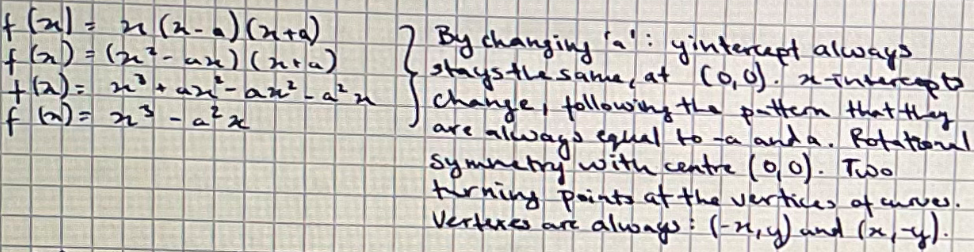For the second family of equations, Sanika wrote:
I noticed that both of them always intersected the $x$ axis at 3 distinct places. When either of them was substituted into the function, it gave a result of $0$ (as $y$ is always zero at the $x$ axis).  I also observed that if a line were drawn parallel to the $x$ axis through the $y$ intercept, the distance from either of the vertices to the line was equal.

This last observation was described differently by Marianna:
The graph appears to have rotational symmetry with the centre being the $y$ intercept.

Marianna, Sanika and Mahdi also found the equations. This is Mahdi's work: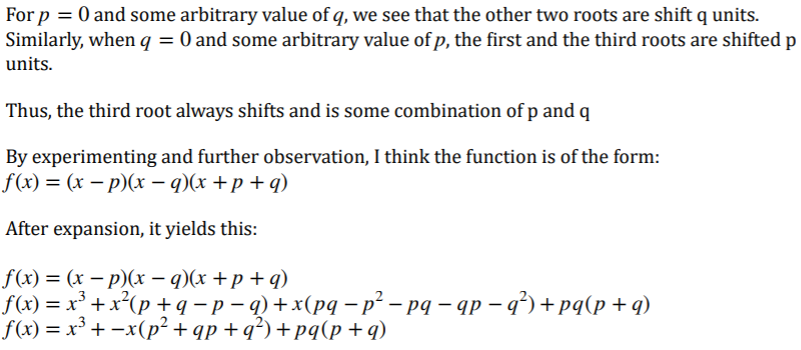Since the sum of the roots is $0,$ the coefficient of $x^2$ will be $0.$

To match the equations to the graphs, Sanika and Marianna tried factorising the cubics and using their roots. This is Sanika's work:

 Graph Equation Factorised cubic 3 distinct roots (1 negative & 2 positive) 3 $(x+1)(x-2)(x-6)$ 1negative root 1 $(x+4)(x2-2x+3)$ 1 positive root 4 $(x-4)(x2+x+3)$ 2 distinct roots (1 negative & 2 positive) 6 $(x-2)(x-2)(x+3)$ 3 distinct roots (2 negative & 1 positive) 2 $(x+3)(x+2)(x-2)$ 2 distinct roots (2 negative & 1 positive) 5 $(x-3)(x+2)(x+2)$

Mahdi used a different relationship between the roots of a cubic and its equation:
In the many functions given, if we assume the three roots to be $\alpha ,\beta ,\gamma$ then any general cubic would be of the form: $$f(x) = x^3 - x^2(\alpha+\beta+\gamma)+x(\alpha\beta+\beta\gamma+\alpha\gamma)-\alpha\beta\gamma$$This comes from expanding $(x-\alpha)(x-\beta)(x-\gamma)$

The key strategy that I followed was first to divide the six graphs into two categories:

$y$ intercept equal to $12$: (A), (B), (D) & (1), (3), (6)

$y$ intercept equal to $-12$ (C), (E), (F) & (2), (4), (5)

The second thing I did was to differentiate all of the equations.

Sang-Ryun from The Perse Upper School in the UK also used differentiation. This is Sang-Ryun's work:
I needed to find the values of the two stationary points that appear in every graph. To achieve this, I used single differentiation for each of the equations.

For example, when considering $y= x^3+2x^2-5x+12$ (equation 1)

$\dfrac{\text{d}y}{\text{d}x}=3x^2+4x-5$

As we are required to find the stationary point of this equation, we know that the gradient of this particular point must equal to $0$ (as tangents to stationary point are always horizontal), we can deduce that in equation 1,

$3x^2+4x-5=0$

We can next use the quadratic formula to solve this equation,

$x=\frac{-b\pm \sqrt{b^2-4ac}}{2a}$

$x=\frac{-4\pm\sqrt{4^2-4\times3\times -5}}{2\times 3}$

To 2 decimal places, the result of this calculation results in,

$x=0.78$ and $-2.12$

However, it is clear that knowing these two $x$ values for the graph is not sufficient, as we are not given the $x$ axis scale within the graphs A - F, and so, we must use the full co-ordinate of the stationary points to accurately determine the relative equations of the graphs, so, I substituted the calculated $x$ values into the original equation (1).

$y= 0.78^3+2\times 0.78^2-5\times0.78+12$
OR
$y=(-2.12)^3+2\times(-2.12)^2-5\times(-2.12)+12$

$y=9.79$ and $22.1$ (3s.f)

We can write the final solution for the two stationary points in co-ordinate notation,

$(0.78, 9.79)$ and $(-2.12, 22.1)$

And now, as we now understand the methodology behind this solution, we can use this same principle for all equations 1 - 6, and I have the results written in hand here: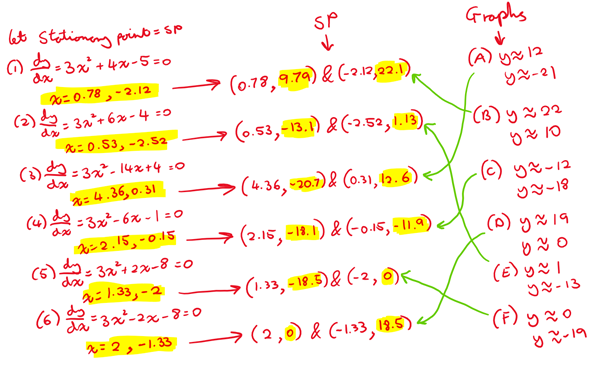And finally...

Muhammad C from Bolton School Boys' Division, Homare from Wimbledon High School (both in the UK), Mahdi and Sanika found the equations of the graphs which make the pattern. Sanika explained how to find the equations:
The first equation $y=(x+6)^3-2$ clearly increases with the value of $x,$ so the graph will start from the bottom and move to the top. The second equation $y=-(x-9)^3+3$ increases when the value of $x$ decreases, so the graph starts from the top and moves to the bottom.

Substituting $x=-6$ into the first equation I get the coordinate pair $(-6, -2).$ This point is seen in the second graph from the left (in the website's illustration). So that must be the graph satisfying the equation. Since all the graphs that increase with the $x$ coordinate is just the graph $y=(x+6)^3 -2$ [translated] along the $x$ & $y$ axes, we can determine the other equations by adding numbers to the $x$ and $y$ components of the equation. The numbers we are adding/subtracting depends on the number of $x$ & $y$ coordinates the graph has shifted. Consecutive graphs increase 1 unit along the $y$ axis and $3$ units along the $x$ axis.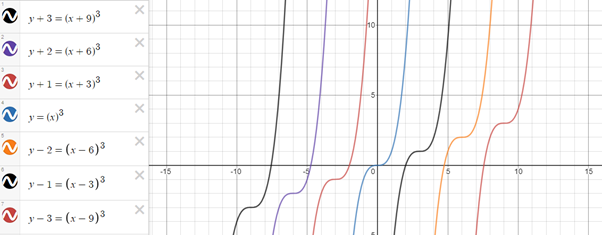The other set of 7 graphs are just the inverse of the above graphs so they would share the same y coordinates but their x coordinates would differ.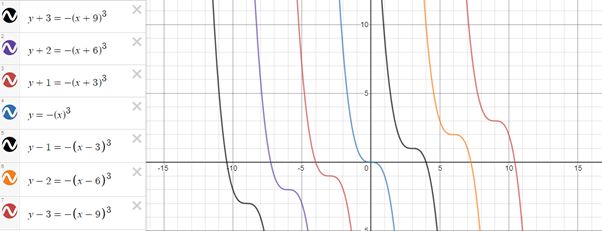As well as these, Homare and Mahdi created some other patterns using cubics on Desmos. These are Homare's patterns:
These are the patterns I made using Desmos! The first being a random pattern I did just to make it look nice. The second is $\frac1{2^k}$ (as the coefficient of $x^3$). The second graph shows how quickly the gradient gets less steep.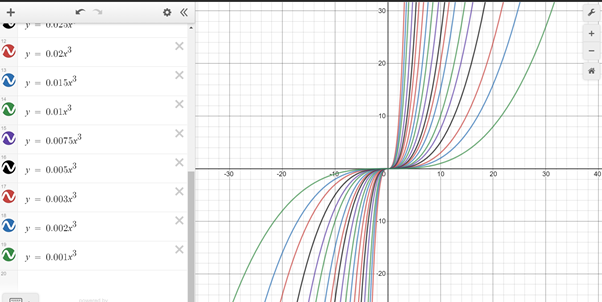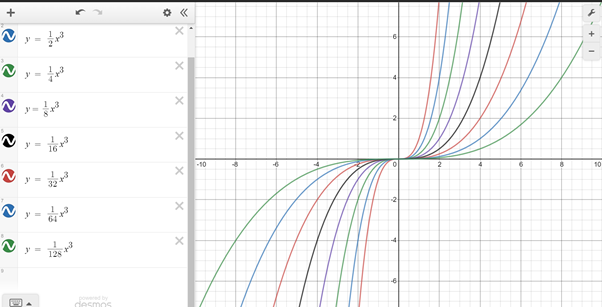These are Mahdi's patterns: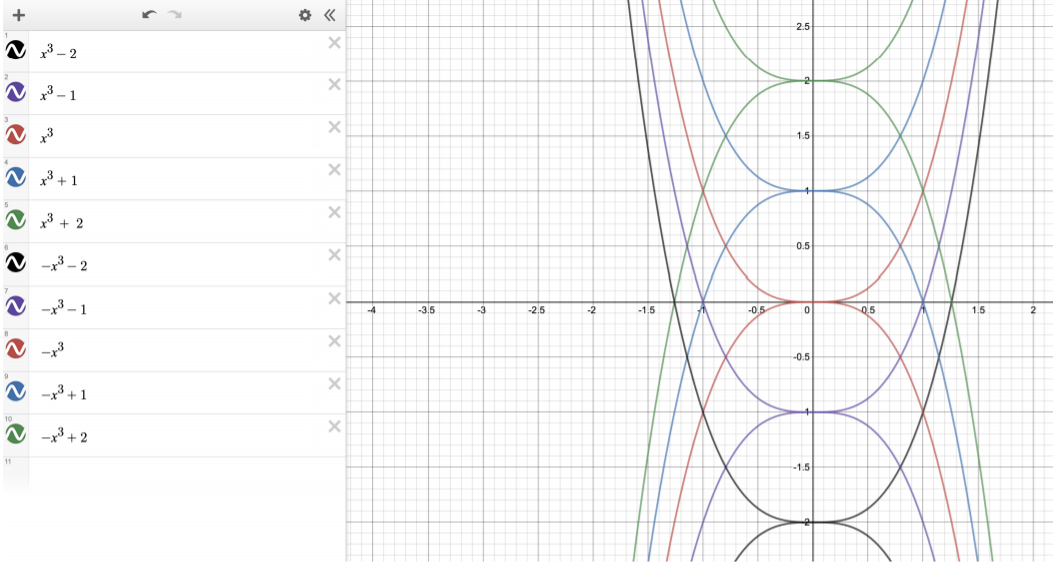Along similar lines (pun intended!), I was able to create one such illustration.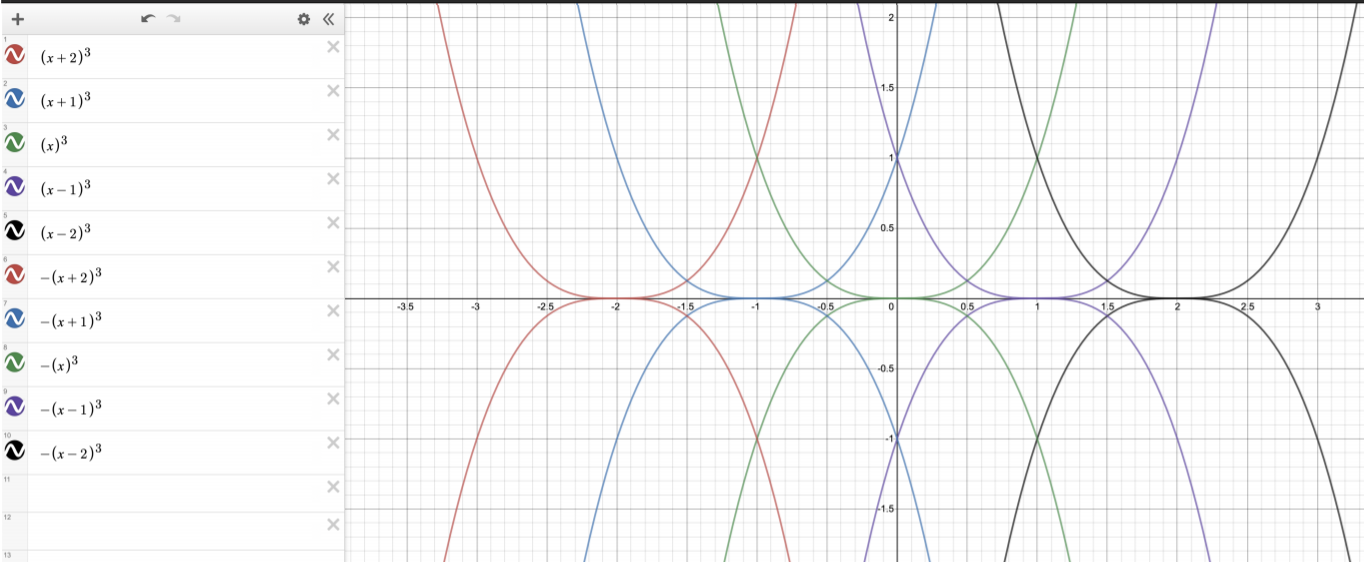By restricting the domain, a pointy star can be created. This can be repeated as well.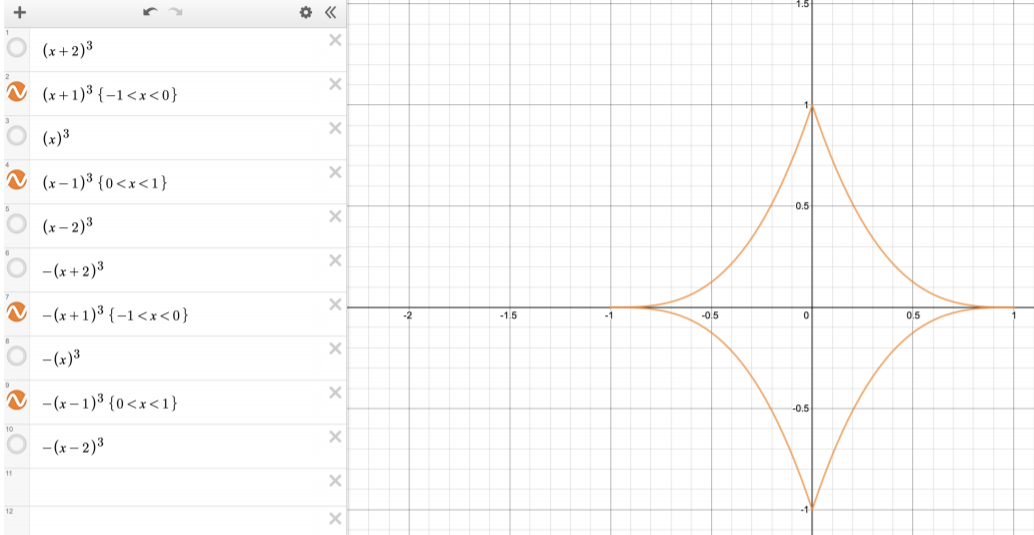I also made a video of multiple cubics harmoniously oscillating around. It was a lot of fun, really enjoyed it.

Click here to see Mahdi's video.